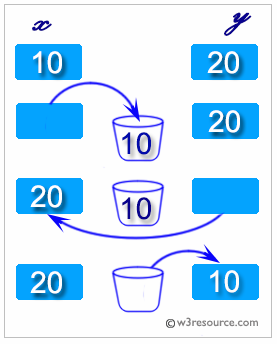# Python: Swap two variables

## Python Basic: Exercise-91 with Solution

Write a Python program to swap two variables.
Python: swapping two variables
Swapping two variables refers to mutually exchanging the values of the variables. Generally, this is done with the data in memory.
The simplest method to swap two variables is to use a third temporary variable :
```define swap(a, b)
temp := a
a := b
b := temp
```Sample Solution:-
a = 30
b = 20
print("\nBefore swap a = %d and b = %d" %(a, b))
a, b = b, a
print("\nAfter swaping a = %d and b = %d" %(a, b))
print()

=========== RESTART: F:/Python_APSC/py-ex-basic-91.py =============

Before swap a = 30 and b = 20

After swaping a = 20 and b = 30

>>>